# 2. Introduction¶

Galaxies. They are some of the most beautiful and fascinating objects in the Universe. They come in a large variety of sizes and shapes, colors and brightnesses, from majestic spirals to seemingly-boring ellipticals, massive cluster members to dinky satellites only seen nearby. Every new way that astronomers devise to look at the sky reveals a different view of galaxies, from the familiar optical and near-infrared images dominated by star light, to the dust emission in the far infrared, and the high-energy processes that shape the far reaches of the wavelength scale. Remarkably, much of the structure of galaxies can be understood using simple physics and this book gives introduction to the quantitative understanding of galactic structure, formation, and evolution that is derived primarily from chasing down the effects of gravity on stellar systems. Of course, while gravity is arguably the most important fundamental force on the scale of galaxies, it does not suffice to understand the full picture of galaxies and their formation and evolution. The physics of radiation, hydrodynamics, nuclear fusion, chemistry, etc. play a fundamental role as well and we will also discuss their important contributions to shaping galaxies.

## 2.1. The world of galaxies¶

Before we start our sojourn in the realm of galaxies, let’s take a quick tour of the types of galaxies found in the Universe. Here are two very beautiful, famous galaxies: M51 and Cen A:

(Credit: M51: NASA/JPL-Caltech/Univ. of Arizona/DSS/SST; CenA: NASA/DOE/Fermi LAT Collaboration, Capella Observatory.)

M51 is a grand-design spiral galaxy. While the origin of spiral structure in galaxies remains an open problem with likely a variety of solutions, in this case the beautiful spiral structure is most probably caused by the response to the gravity from the companion galaxy at the top of the image. Spiral structure, besides looking pretty, is an important driver of star formation, as the gravitational force of the spiral compresses gas, leading to the formation of new stars within the spirals. These show up as the red dots in the image above, which are small clusters of young stars (traced by their ionized HII emision here).

Cen A is one of the brightest galaxies in the sky and an example of an S0 galaxy, a galaxy somewhere between the extremes of elliptical and spiral galaxies. Its exact classification is still a matter of debate and this illustrates the difficulty we face when studying galaxies. The only data we have is a single snapshot in time from a single perspective, with very limited information on its three-dimensional structure. Because no galaxy is a fully relaxed system, all galaxies are evolving and many are going through dramatic transformations. Cen A has probably endured a merger in its recent past, leading to a burst of star formation and the fueling of an active galactic nucleus (AGN)—a supermassive black hole with a bright accretion disk—at its center.

While most of the famous galaxies are as large as those pictured above, many other galaxies are quite a bit smaller. We find many examples of these in our own backyard, as satellite galaxies of the Milky Way. Here are two examples: the Large Magellanic Cloud (LMC) and the Fornax dwarf galaxy (not to be confused with the Fornax cluster!):

(Credit: LMC: Dylan O’Donnell; Fornax: ESO/Digitized Sky Survey 2)

The LMC is one of the closest neighbors of the Milky Way and the largest of its satellite galaxies—galaxies caught in the Milky Way’s gravitational attraction. The LMC is part of a pair of galaxies that fell into the Milky Way’s gravitational field together and its irregular appearance is in large part due to the tidal interactions with its sibling, the Small Magellanic Cloud, and with the Milky Way. The LMC has a prominent bar at its center and was likely a barred spiral galaxy before it started interacting with the Milky Way. The LMC is about 10 times smaller in size than the Milky Way and somewhere between 10 and 100 times smaller in mass. It has lots of gas and many areas of active star formation. Some of the most famous star-forming regions are in the LMC.

Fornax is an example of a dwarf spheroidal galaxy, which are the smallest galaxies. Such galaxies are much more diffuse, as can be seen by comparing the Fornax dwarf galaxy to the pictures of M51 or Cen A above, and their masses are dominated by dark matter, making them some of the best places we have to constrain the fundamental nature of dark matter. Fornax in particular is a bit of a dynamical celebrity, because it has a number of globular clusters. If the dark-matter profile in the centers of galaxies has the expected, cuspy shape, then the process of dynamical friction should have caused these clusters to have long since spiraled into the center (Tremaine 1976). That Fornax still has these globular clusters therefore indicates that the dark-matter density is lower than expected at its center.

Circling back to Milky-Way-sized galaxies for the moment, here are two more examples of large disk galaxies: NGC 1300 and M81:

(Credit: NGC1300: NASA, ESA, and The Hubble Heritage Team STScI/AURA); M81: NASA, ESA and the Hubble Heritage Team (STScI/AURA))

The most obvious property of NGC 1300 is the rectangular-ish feature at its center, with two prominent spiral arms coming off of its sides. This feature is called a bar (for obvious reasons) and it is a common feature of disk galaxies. Overall, about 30% of disk galaxies in the local Universe have bars, a fraction that goes down as we look further into the past, showing that bars are a relatively recent (in cosmic time units!) feature of galaxies. Our own Milky Way has a bar at its center, the exact properties of which are still up for debate, but it is far less strong than the bar in NGC 1300. Bars form naturally during the evolution of disk galaxies.

M81 is a spiral galaxy without an obvious bar at its center. Instead it has a prominent bulge, a spheroidal concentration of mostly old stars, and tightly wound spirals. M81 is part of a group of galaxies much like the Local Group, the set of galaxies that includes the Milky Way and the Andromeda galaxy (which we will typically refer to as M31), their satellites, and some more small galaxies associated with them. M81 is interacting with M82 and NGC 3077, a good reminder that almost no galaxy lives in isolation.

We could keep showing pictures of barred and spiral disk galaxies (and of barred-spiral disk galaxies!), but the following is an example of an elliptical galaxy: NGC 3923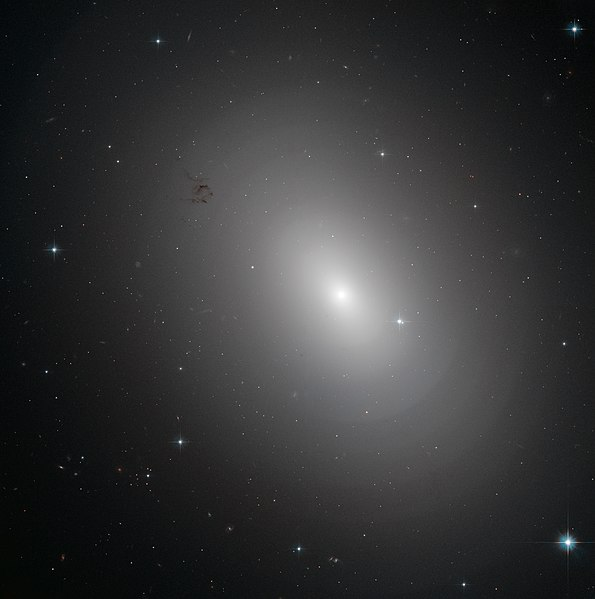(Credit: NASA/ESA Hubble Space telescope)

NGC 3923 is a more-interesting-than-usual example of an elliptical galaxy, which normally have smooth, elliptical light distributions without any features. Even though most elliptical galaxies might at first glance therefore seem a little boring, their internal orbital structure can be quite complex. NGC 3923 is especially interesting, because it has multiple shell-like features in its outer parts; such galaxies are known as shell galaxies. This indicates that the stars are arranged in a structure more akin to layers than in a smooth, continuous distribution like in other elliptical galaxies. Such shell-like features are common in elliptical galaxies, with an estimated 50% of elliptical galaxies displaying faint shells. These shells are an indication that NGC 3923 has experienced a merger with a small satellite and the distribution of orbits of stars in the satellite got re-arranged to give rise to a density profile with sharp edges.

Galaxies are not just made up of single stars, but also contain stellar clusters of various sorts. Because the evolution of these clusters is intimately related to the evolution of their host galaxies, we will also give them some attention in this book. Two of the main types of stellar clusters are globular clusters and open clusters. Here is an example of one of each: the globular cluster M80 and the open cluster M11:

(Credit: M80: NASA, The Hubble Heritage Team, STScI, AURA; M11: NASA)

It is immediately clear that these are two quite different beasts. Globular clusters are extremely dense. The center of M80 is a blob of light, even in this picture taken by the Hubble Space Telescope, because there are too many stars close together to be able to resolve all of them as individual stars in this image. This particular globular cluster, M80, is one of the most massive of the $$\approx 150$$ globular clusters in the Milky Way. Globular clusters are old, found in almost all galaxies, and consist of thousands to hundreds of thousands of stars. Because they are so dense, globular clusters display a rich dynamical phenomenology that is quite different from that of galaxies. Dynamics and stellar evolution are also intimately linked in globular clusters, making them a fine laboratory for stellar evolution and potentially factories for binary black holes such as those seen by LIGO. Globular clusters can also get disrupted by tidal interactions with their host galaxies, leading to the production of narrow stellar streams.

Open clusters are very different from globular clusters. The main difference is that they are much less dense and many of them are only marginally bound. Open clusters dot the disks of star-forming galaxies. They consist of stars born together in a (typically recent) star-formation event. Open clusters are typically only a few hundred millions years old, although some survive for many billions of years. The open cluster pictured above, M11, is about 250 million years old and is a rich open cluster with about 3,000 stars. Open clusters are an important laboratory for simple stellar evolution (because they are less dense than globular clusters, their internal dynamics does not mix with their stellar evolution). Open clusters get easily disrupted by tidal forces and only the most tightly bound therefore survive for billions of years.

## 2.2. Brief tour of galaxy observations¶

To set the stage for the material covered in this book, we start with a high-level overview of the various components that make up galaxies. The objective here is not to provide a detailed and exhaustive overview of galaxy phenomenology, but merely to briefly discuss the size, shape, and contribution to the overall mass budget of different galactic components. The next chapters will then describe how we calculate the gravitational potential corresponding to the total mass distribution and use this to investigate the structure and mass distribution of galaxies. We will add more detailed discussion of the structure of different galaxy types as we go along in our exploration of galaxies.

We will focus our overview on large disk galaxies. Large disk galaxies are one of the most important type of galaxies for various reasons: approximately half of all galaxies in the local Universe are disk galaxies and such galaxies appear to be most efficient at converting gas into stars. Therefore, most stars in the local Universe live in the disks of large disk galaxies (even though smaller galaxies are more numerous, they also contain many fewer stars, so at the end of the day, there are more stars in large disk galaxies). We also live close to the midplane of a large disk galaxy, the Milky Way. This provides us with a detailed, close-up look of the structure of such a galaxy. In the Milky Way, we can observe stars from the most luminous giants to the lowest mass M dwarfs, from the center of the Galaxy to the outskirts of the spherical stellar halo surrounding the disk, and we can study gas in its various atomic and molecular phases. Because galactic disks are “dynamically cold”, meaning that the ratio of their velocity dispersion to the typical velocity of a star is small, they are also prone to instabilities that give rise to bars, spiral structure, etc., which are phenomena that we will discuss in later chapters.

Here is a picture of a typical disk galaxy, NGC 4565, seen edge-on: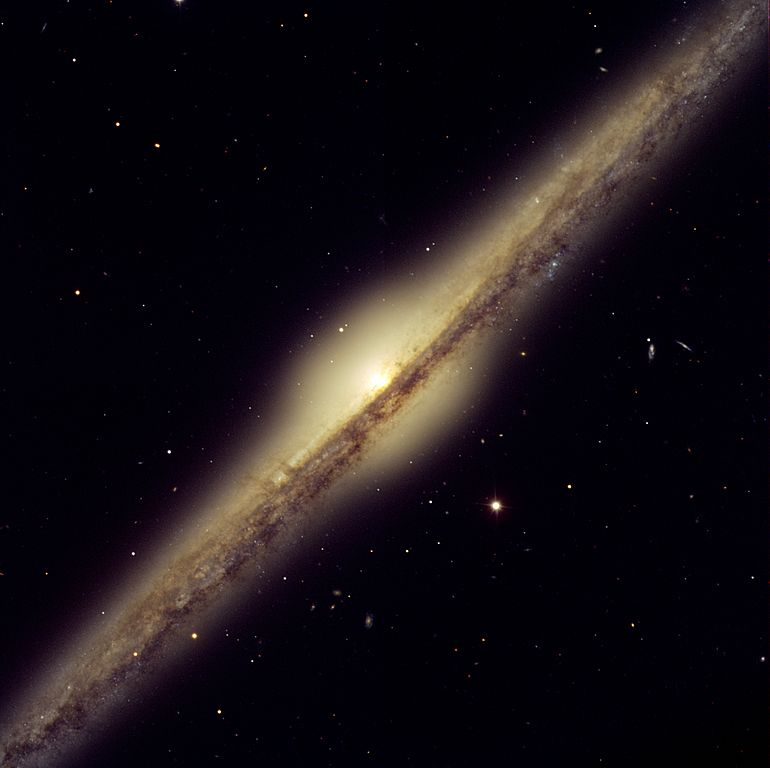(Credit: ESO)

NGC 4565 displays all of the components of a typical disk galaxy. Its structure is dominated by a disk made up of stars and gas, with a protusion of stars near the center that is called the bulge. The gas itself cannot be seen in this picture, but its presence can be inferred because gas is traced by dust grains, which redden passing starlight and cause the reddish band close to the midplane of the disk. The stars surrounding the disk are foreground Milky Way stars unassociated with NGC 4565. NGC 4565 does have a stellar halo (Harmsen et al. 2017), but it is too faint to be seen in this picture. Also not seen in this picture (and not seen directly—whatever that means—in any picture of any galaxy) is the spheroidal distribution of dark matter that surrounds the disk and extends to very large distances from the center.

Here is a schematic overview of a disk galaxy, the Milky Way in particular (but except for the Sun’s position, this applies to all disk galaxies):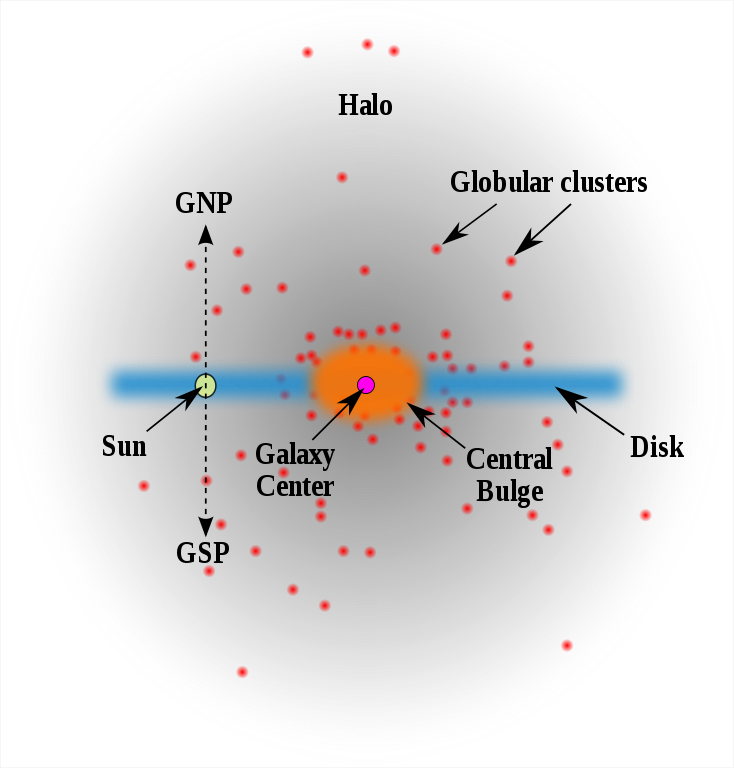(Credit: RJHall at English Wikipedia)

This illustration shows the disk edge-on and displays the system of globular cluster that surrounds each galaxy in addition to the components that we already discussed. The Galactic center is also separately emphasized. We’ve known for about two decades that the centers of galaxies host supermassive black holes (see Chapter 17), which can be active and surrounded by an accretion disk (such accretion disks are a topic that we will not cover in this book) or inactive and often surrounded by a dense nuclear star cluster. The dynamics of these star clusters are interesting because they are dense and they consist of relatively few stars (compared to the entire galaxy), which leads to interesting new dynamical phenomenology. This setup is similar to that of globular clusters, except for the supermassive black hole, which has a large effect on the ensuing dynamics. But we are getting off topic!

### 2.2.1. The distribution of stars in galaxies¶

One of the most basic observations about the disks of galaxies is that they are exponential. That is, their light profiles $$I(R)$$ follow an exponential decline with radius:

\begin{equation} I(R) = I_0\,\exp\left(-R/h_R\right)\,. \end{equation}

This had been known in the mid-1900s, but was most famously discussed by Freeman (1970) “On the disks of spiral and S0 galaxies” (oh, to be able to write a paper with a title like that!). The following is the first figure in Freeman’s paper, which shows the surface brightness as a function of radius along the major axis in the blue B band: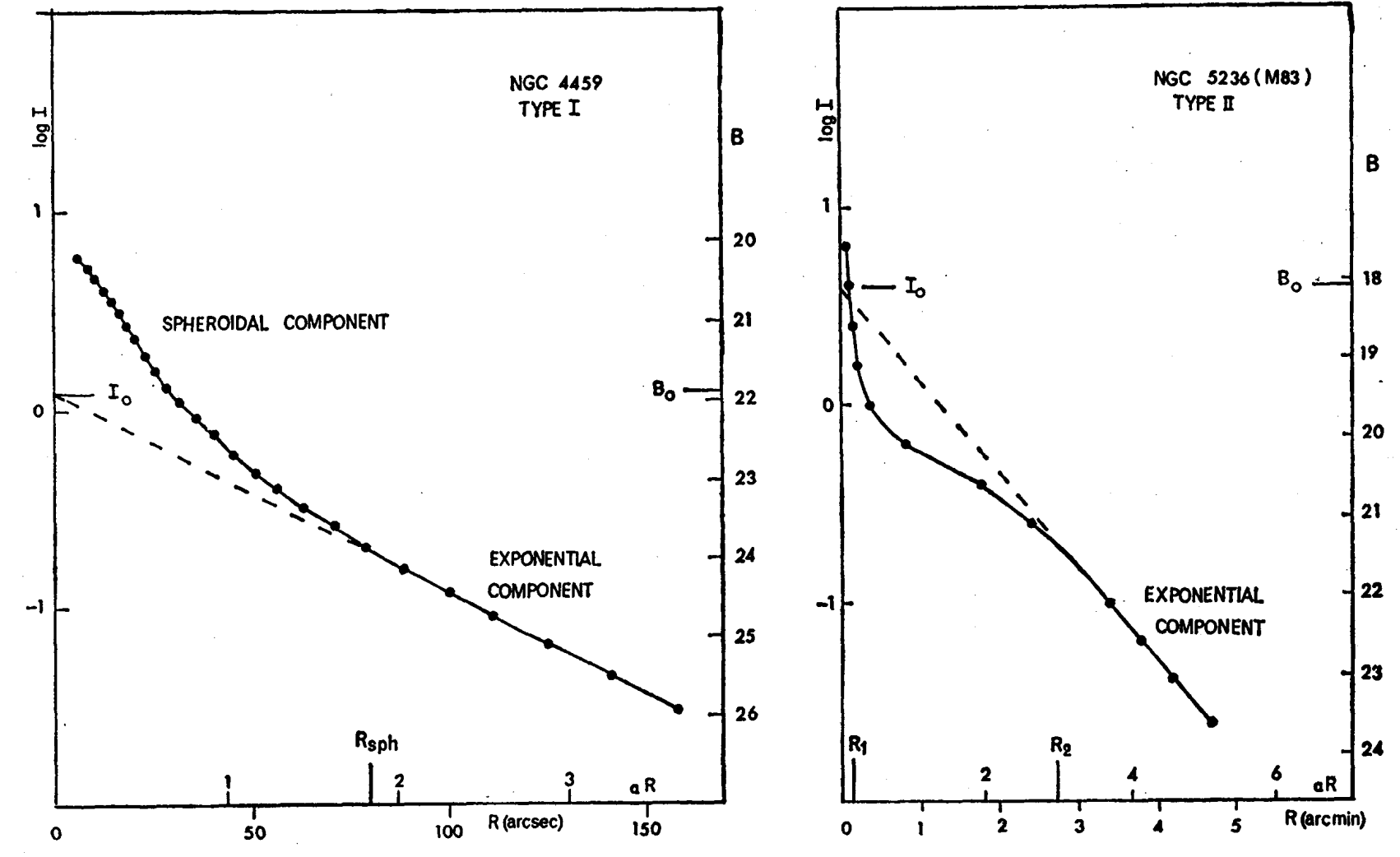(Credit: Freeman 1970)

Because surface brightness is given on the y axis as a logarithmic quantity measured in magnitudes, the linear decline implies an exponential decline in actual surface brightness. Both of the shown galaxies only follow the exponential law over a restricted radial range. Near the center they rise precipitously, due to the presence of the bulge (the “spheroidal component” above); the galaxy on the right also has a flattened part of the light profile near the center, which is not uncommon.

The second most important dimension of a disk is its vertical dimension. Vertical for the moment means perpendicular to the two-dimensional plane of the disk, the direction along which the disk is narrowest (in Chapter 8 we will define the vertical direction as the direction of the angular momentum vector of the disk). Along the vertical direction, the density also falls off quickly in a roughly symmetric manner; the peak of the vertical density occurs at the midplane, the zeropoint of the vertical coordinate. Like for the radial profile, we look at the vertical profile of the surface brightness to get a sense for what the vertical mass distribution is. The following is a typical result: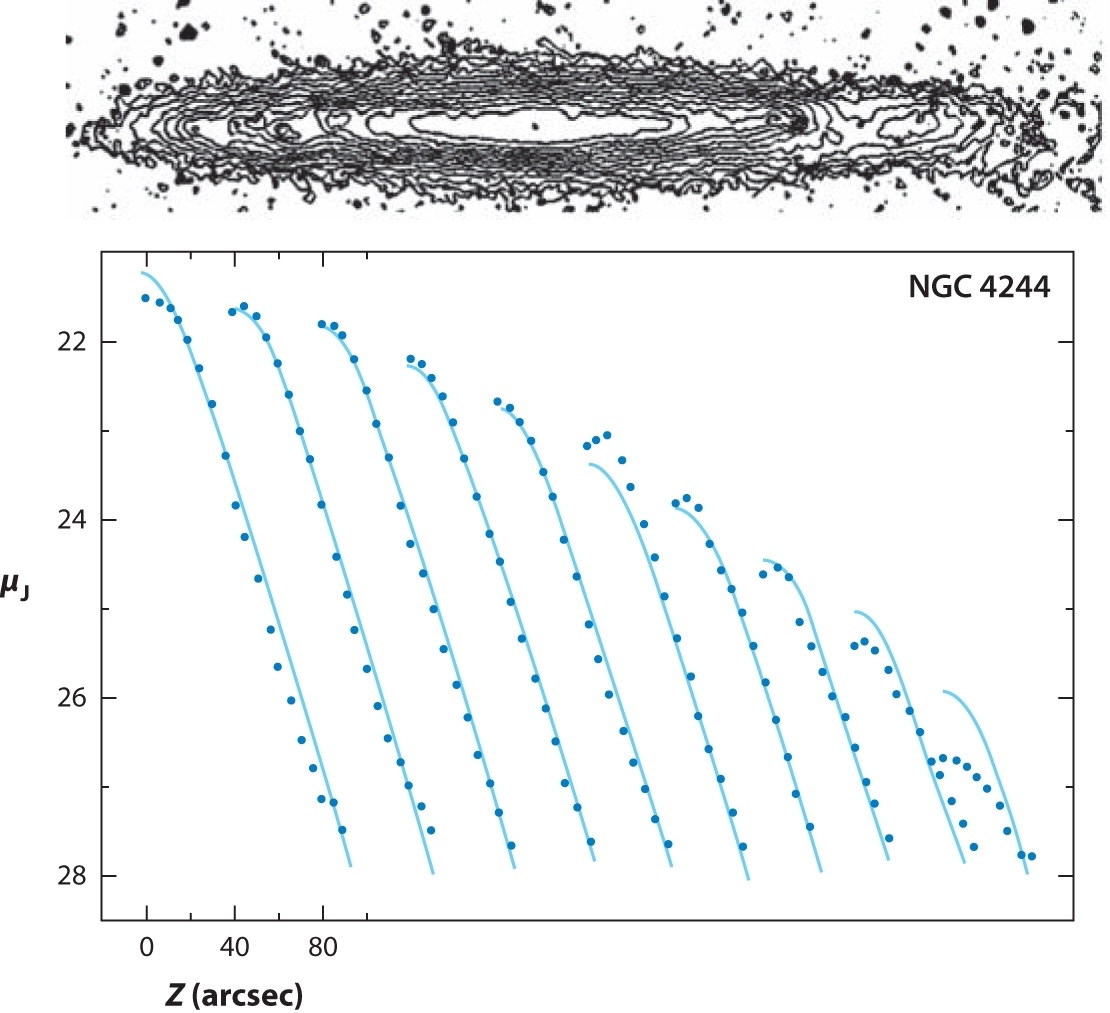(Credit: van der Kruit & Freeman 2011; NGC 4244: top: a pure-disk galaxy seen edge-on; bottom: vertical surface-brightness profiles at a range of distances from the center [profiles are displaced along the X axis to avoid overlap])

This figure shows vertical surface-brightness profiles for NGC 4244 at different distances from its center. Again we see a linear decline at large distances, which implies an exponential fall-off (because the surface-brightness in this figure is a logarithmic quantity). Near the midplane the profile flattens and becomes close to constant. The fit that is shown is a $$\mathrm{sech}^2$$ profile, a hyperbolic secant squared, which is the equilibrium solution of a self-gravitating isothermal disk as we will discuss in Chapter 11.4. As we discussed above and will discuss below in more detail, disks contain gas and stars of various ages with different vertical distributions and the simple isothermal—having the same amount of random motion at each vertical height—can therefore not be entirely correct. The $$\mathrm{sech}^2$$ profile should therefore not be taken too seriously, but is simply a physically-motivated profile that does a good job of fitting the observations.

The ratio between the scale length $$h_R$$ and the scale height $$h_z$$ is a measure of the thickness of the disk. A typical value is $$h_R / h_z \approx 10$$, meaning that disks are quite thin.

Whether we use number counts or surface brightness, we have to translate these to mass profiles by assuming stellar-population models for how mass is traced by number counts or surface brightness. This is done by applying a mass-to-light ratio $$M/L$$ that converts the amount of light observed to an amount of (stellar) mass and is usually expressed in solar units (the Sun’s mass over the Sun’s luminosity). The stellar mass-to-light ratio is typically assumed to be constant through the galaxy and has typical values of $$M/L \approx 3$$, but it depends on the passband in which the luminosity is measured. The total mass-to-light ratio in galaxies is much larger and depends on position, because in addition to stars it includes the dark matter, which adds mass, but does not contribute to the luminosity; the total $$M/L$$ also includes gas, which in broad optical and near-infrared passbands similarly does not contribute to the luminosity, while adding mass (but generally much less mass than either stars or dark matter; see below). The total mass-to-light ratio depends on position because the fraction of total mass that is dark matter and gas is strongly dependent on position.

While the radial profile of the disk is (close to) exponential, the central bulge is not. Bulges typically have profiles similar to elliptical galaxies, which are modeled as Sérsic profiles:

\begin{equation}\label{eq-sersic} \mu(R) = \mu_0 - b_n R ^{1/n}\,, \end{equation}

where $$\mu$$ is the surface brightness, $$\mu_0$$ is the central surface brightness, $$n$$ is the Sérsic index, and $$b_n$$ can be computed from the value of $$n$$. The case of $$n=4$$ is the classic de Vaucouleurs profile. $$n=1$$ is an exponential profile.

As an example, here is the surface-brightness profile of M31, the closest large disk galaxy outside of the Milky Way: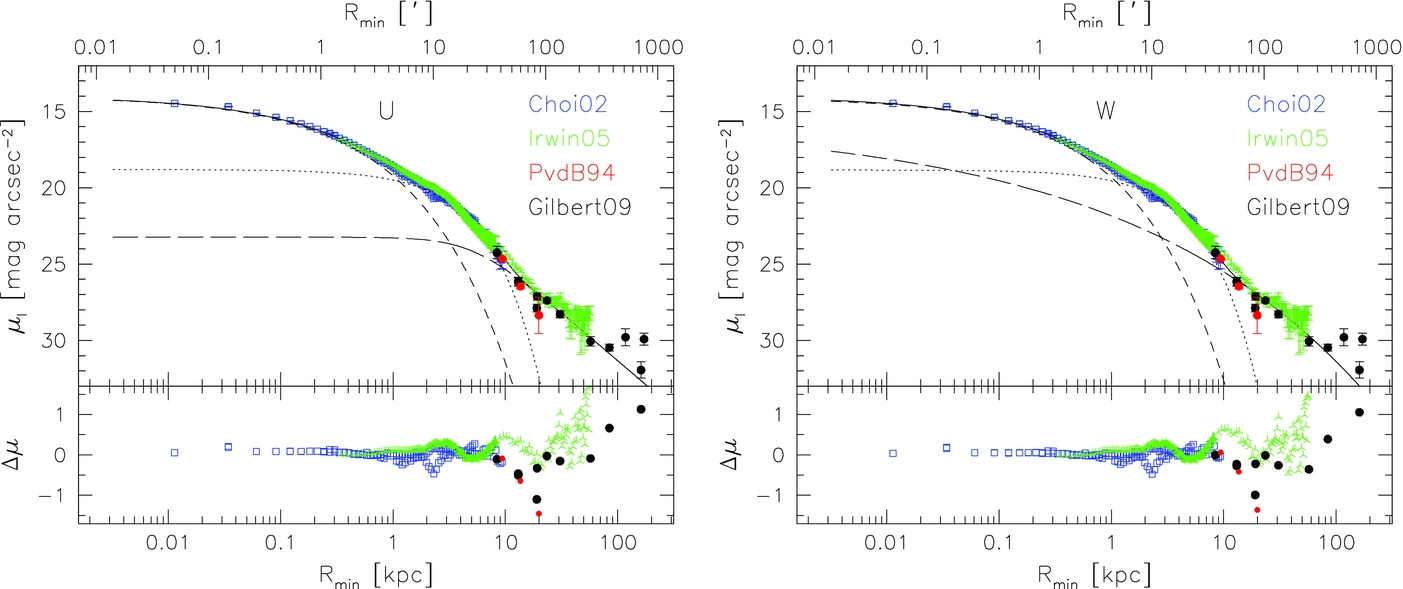(Credit: Courteau et al. 2011; each panel shows the surface-brightness profile of M31 along with a fit with a Sérsic bulge (dashed), exponential disk (dotted) and halo (long dashed) with the combined fit profile as the full curve; halo model is a power-law on the left and Sérsic on the right; bottom panels: fit residuals).

M31’s bulge component is a dashed line in fits to this profile with a Sérsic bulge, exponential disk, and a stellar halo (with two different radial profiles in the two panels). The bulge has a Sérsic index of about 2.2. The disk, given by the dotted line, starts to dominate the light outside of 1 kpc. The following figure directly shows the fraction of the light at each radius contributed by the bulge, disk, and stellar halo:(Credit: Courteau et al. 2011).

The bulge dominates within 1 kpc, the very central region of the galaxy, the disk then dominates out to 10 kpc, and the stellar halo dominates the light outside of the disk region. Given how prominent the stellar halo is outside of the disk region, let’s take a closer look at this component. The following figure shows deep observations of some external disk galaxies. These observations are deep enough to reveal the low surface-brightness envelope surrounding these disks (the actual disks are painted in from shallower observations):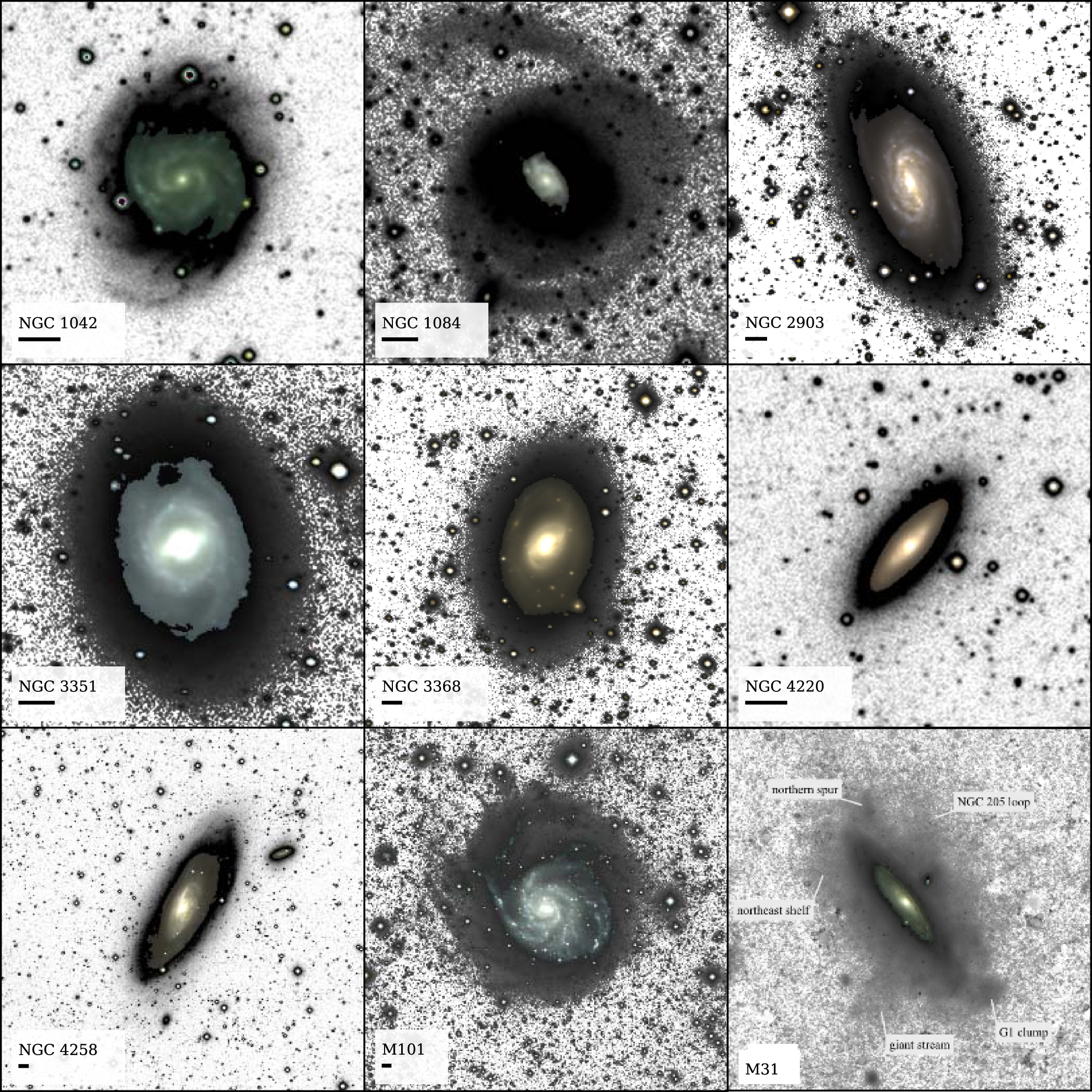(Credit: Merritt et al. 2016).

The following figure displays the actual radial surface-brightness profile for these galaxies (the actual y axis shows the logarithm of the surface density, because the authors have assumed a mass-to-light ratio; don’t be fooled into thinking that they measured actual mass!):(Credit: Merritt et al. 2016).

The dashed line in all of these panels shows an exponential-disk fit to these galaxies (in addition to a central bulge); it’s a straight line in this space. Many of these galaxies have excess light at large radii (> 20 kpc) beyond this exponential disk. This light extends in a spheroidal manner, not a disk, and follows an approximate power-law profile. It is clear from this figure that there is great diversity in the profiles of the stellar halo. This is because the stellar halo is believed to form primarily from mergers (accreted material from merging satellite galaxies, or material from the main galaxy disturbed by a merger).

While the stellar halo takes up a large volume, its total stellar mass is much smaller than that of the other main galactic components. The stellar halo typically only accounts for a few percent of the stellar mass of a galaxy and much less than that of the total mass. There is no part of a galaxy where the stellar halo dominates the density, because it is always overwhelmed by either the bulge, disk, dark-matter halo, or the gaseous component.

### 2.2.2. The distribution of gas in galaxies¶

All baryonic matter was once in gaseous form, but in large, present-day disk galaxies only about 10% of the baryonic mass is present as cold/warm gas in the interstellar medium. Gas is present in galaxies in different phases and in both atomic and molecular form. Making a precise census of all of the gas in galaxies is difficult. In particular, determining the amount of hot gas in the halo is very difficult.

Stars form from cold molecular gas. Cold gas is contained in a disk component much like the stellar disk component that we discussed above. Most of the gas is atomic hydrogen, or HI. The following shows the cold-gas content (HI in particular) as a function of stellar mass for a sample of galaxies in the local Universe: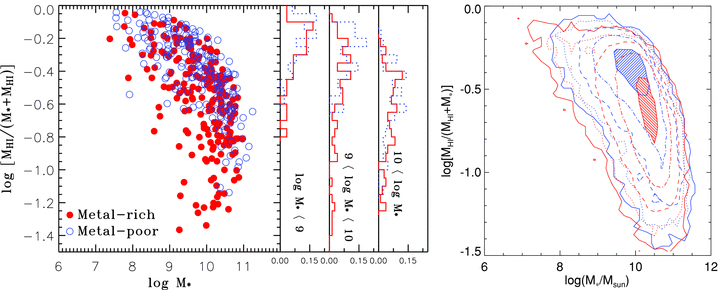(Credit: Zhang et al. 2009; left panel: HI gas mass fraction vs. stellar mass for metal-rich (red) and metal-poor (blue) galaxies, for galaxies with direct HI observations; right panel: HI mass fraction vs. stellar mass for star-forming galaxies with indirect HI measurements.)

The Milky Way’s stellar mass is about $$6 \times 10^{10}\,M_\odot$$ or $$\log (M_*/M_\odot) \approx 10.8$$; gas makes up about 10% of the baryonic disk mass in the Milky Way. The figure above shows that the gas fraction rises as one goes to lower mass galaxies and the dynamics of gas and its interaction with stars is thus much more important in lower mass galaxies than it is in the Milky Way.

In the Milky Way, we can make a reasonably complete census of the interstellar medium near the Sun. The main components are: atomic hydrogen (HI), ionized hydrogen (HII), and molecular hydrogen ($$H_2$$). The following table gives their local densities and vertical distribution, as well as the integrated column density perpendicular to the disk plane:(Credit: McKee et al. 2015)

Converting the number density in the midplane from the table above to a mass density, we find that the midplane gas density is about $$0.041\,M_\odot\,\mathrm{pc}^{-3}$$, approximately the same as that in stars ($$\approx 0.040\,M_\odot\,\mathrm{pc}^{-3}$$; Bovy 2017b). The molecular gas layer has an exponential scale height that is approximately $$h_z = 100\,\mathrm{pc}$$, the coldest component of HI has about the same thickness, while the ionized gas is contained in a much thicker layer.

The radial distribution of these components is much less certain. The radial surface density of HI is shown in the following figure: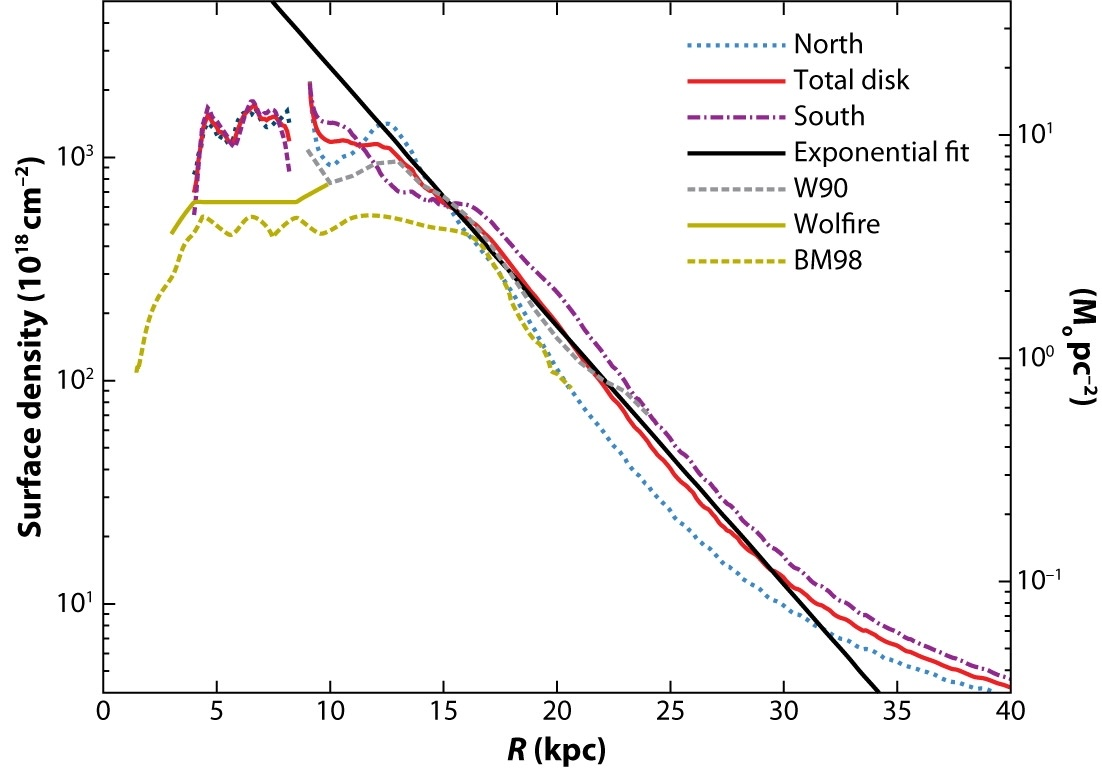(Credit: Kalberla & Kerp 2009)

Thus, the density of HI is almost constant within 10 kpc or at most displays a shallow decline; outside of 10 kpc it falls off exponentially. But note that there are significant asymmetries between different parts of the distribution.

A smaller fraction of the ISM is contained in molecular gas (about 10% near the Sun). The radial profile of the molecular gas is similar to that of the HI: it is approximately constant in the inner Galaxy and drops off exponentially at larger radii: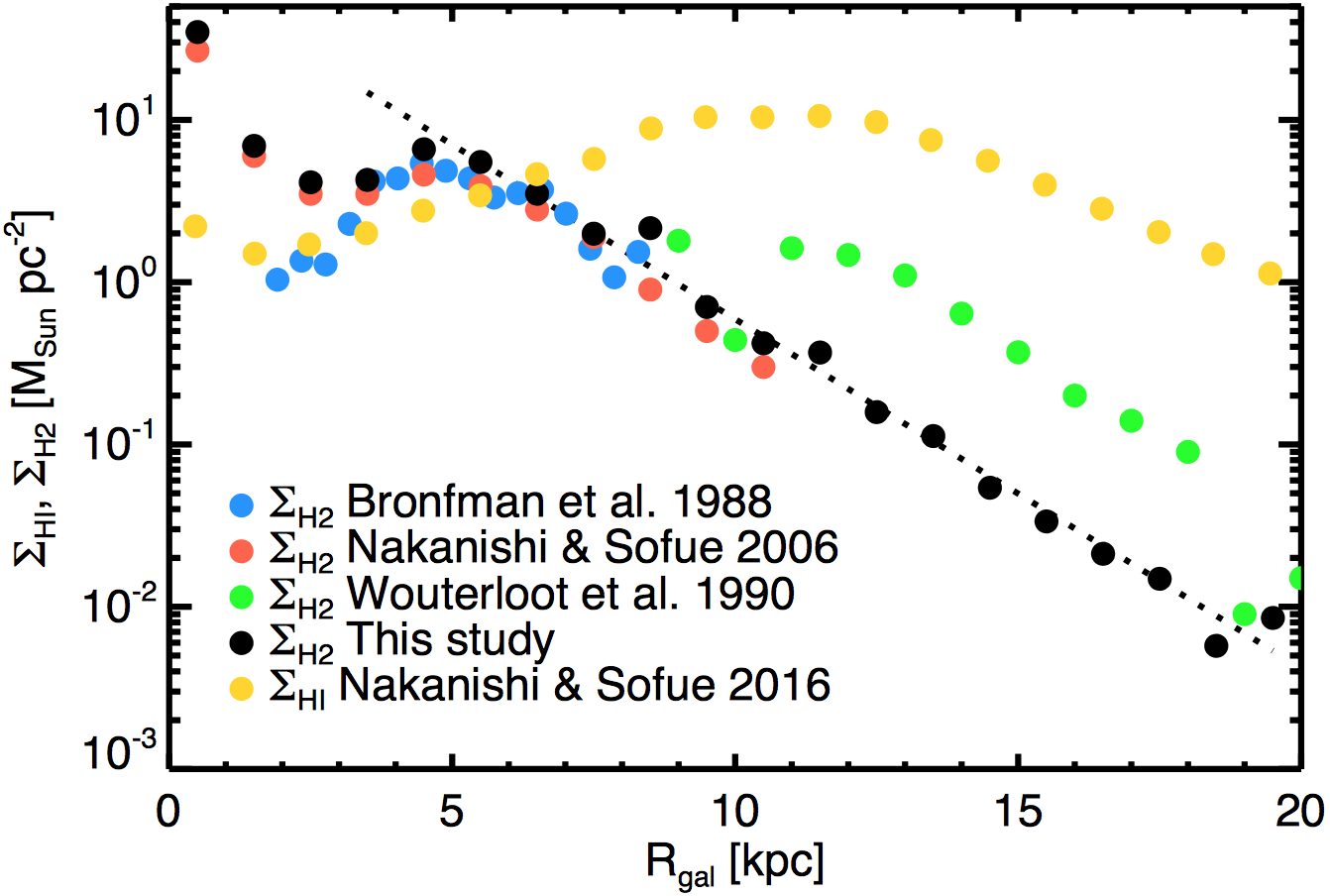(Credit: Miville-Deschênes et al. 2017)

But the molecular gas has a shorter scale length than the HI, so very little of it is found outside of 15 kpc. Almost all of the molecular gas is contained in molecular clouds.

The figure above was made from a recent molecular-cloud catalog. We load this catalog from Miville-Deschênes et al. (2017) in the following interactive figure:

This figure shows the molecular clouds in the disk plane of the Milky Way as seen from above, with the Sun at $$(X,Y) = (8,0)$$ kpc. The area of the points is proportional to the total mass of each cloud (with a simple linear scaling) and the points are color-coded by their velocity with respect to the Sun. You can zoom in and out to get a closer look at the distribution. It is clear that most of the large clouds are located closer to the center than the Sun is. The velocity pattern is also clearly not random! What creates this pattern of velocities? After reading Chapter 9 you should be able to answer this question. The answer isn’t as obvious as you might think!

### 2.2.3. The distribution of mass in galaxies¶

Now that we have a good sense of the stellar and gas distribution in a typical disk galaxy, let’s take a look at the largest contributor to the galactic mass distribution: the dark-matter halo. Dark matter is a largely unknown constituent of the Universe, likely a new particle, that overall is about 5 times more abundant (e.g., Planck Collaboration et al. 2016) than ordinary matter (sometimes called “baryonic matter” although this misses the ordinary, fermionic matter that is, however, negligible in mass compared to its baryonic counterpart). In galaxies such as the Milky Way there is more than ten times more dark matter than ordinary matter, with the remaining baryonic matter likely in warm and hot phases in the interstellar and intergalactic medium (e.g., Shull et al. 2012). We know about the presence of dark matter solely through its gravitational influence and this book will cover how we have learned and are continuing to investigate the distribution of dark matter in galaxies.

To get a sense of the distribution of dark matter, we will plot the density and enclosed mass of different components of the Milky Way. We use the mass model for the Milky Way from McMillan (2017). This model has a bulge, disk, and dark-matter halo potential (ignoring the contributions from the stellar halo and including those from the gas together with the stellar disk). First we look at the density:

:

from functools import reduce
from galpy import potential
from galpy.potential.mwpotentials import McMillan17
from galpy.util import plot as galpy_plot
from matplotlib.ticker import FuncFormatter
from astropy.visualization import quantity_support
figsize(10,10)
rs= 10.**(numpy.linspace(-1.,2.5,101))*u.kpc
subplot(2,1,1)
# Bulge
line_bulge= galpy_plot.plot(\
rs,
McMillan17.dens(rs,0.5*u.kpc),
lw=2.,label=r'$\mathrm{Bulge}$',loglog=True,
ylabel=r'$\rho(r,z=0)\,(M_\odot\,\mathrm{pc}^{-3})$',
xrange=[0.1,400.],yrange=[10.**-5.7,10.],gcf=True)
# Disk
line_disk= loglog(rs,
McMillan17.dens(rs,0.5*u.kpc),
lw=2.,label=r'$\mathrm{Disk}$')
# DM halo
line_dm= loglog(rs,
McMillan17.dens(rs,0.5*u.kpc),
lw=2.,label=r'$\mathrm{Dark\ matter\ halo}$')
# Full
alldens= [reduce(lambda x,y: x+y,[pot.dens(r,0.5*u.kpc)
for pot in McMillan17]) for r in rs]
alldens= numpy.array([d.to(u.solMass/u.pc**3).value for d in alldens])\
*u.solMass/u.pc**3
line_full= loglog(rs,alldens,
color='k',lw=2.,label=r'$\mathrm{Combined}$')
legend(handles=[line_bulge,line_disk,line_dm,line_full],
fontsize=18.,loc='upper right',frameon=False)
gca().xaxis.set_major_formatter(FuncFormatter(
lambda y,pos: (r'${{:.{:1d}f}}$'.format(int(\
numpy.maximum(-numpy.log10(y),0)))).format(y)))
# Panel with relative density
subplot(2,1,2)
# Bulge
line_bulge= galpy_plot.plot(\
rs,
McMillan17.dens(rs,0.5*u.kpc)/alldens,
lw=2.,label=r'$\mathrm{Bulge}$',semilogx=True,
xlabel=r'$r\,(\mathrm{kpc})$',
ylabel=r'$\rho_i(r,z=0)/\rho_\mathrm{total}(r,z=0)$',
xrange=[0.1,400.],yrange=[0.,1.1],gcf=True)
# Disk
line_disk= semilogx(rs,
McMillan17.dens(rs,0.5*u.kpc)/alldens,
lw=2.,label=r'$\mathrm{Disk}$')
# DM halo
line_dm= semilogx(rs,
McMillan17.dens(rs,0.5*u.kpc)/alldens,
lw=2.,label=r'$\mathrm{Dark\ matter\ halo}$')
legend(handles=[line_bulge,line_disk,line_dm],
fontsize=18.,loc='upper left',frameon=False)
gca().xaxis.set_major_formatter(FuncFormatter(
lambda y,pos: (r'${{:.{:1d}f}}$'.format(int(\
numpy.maximum(-numpy.log10(y),0)))).format(y)))
tight_layout();The top panel shows the actual density of the different components in the midplane of the Milky Way (at $$z = 0$$) as well as the total density, while the bottom panel shows the relative density of the three main different components. Much like in M31 above, the bulge component dominates the density within 1 kpc, but becomes less important after that. The stellar disk dominates the density between 1 kpc and about 10 kpc, after which the dark-matter halo dominates.

The total density near the Sun (at 8 kpc from the center) is about $$0.1\,M_\odot\,\mathrm{pc}^{-3}$$, with only about 1/10th of that contributed by dark matter. The following figure shows the total mass enclosed within a given radius for the different components and for the total mass:

:

figsize(10,6)
rs= 10.**(numpy.linspace(-1.,2.5,31))*u.kpc
# Bulge
bulge_mass_enc= numpy.zeros(len(rs))*u.solMass-1.*u.solMass
bulge_mass_enc[rs < 10.*u.kpc]= \
numpy.array([McMillan17.mass(r).to(u.solMass).value
for r in rs if r < 10.*u.kpc])*u.solMass
bulge_mass_enc[rs >= 10.*u.kpc]= numpy.amax(bulge_mass_enc)
line_bulge= galpy_plot.plot(rs,bulge_mass_enc,loglog=True,
lw=2.,label=r'$\mathrm{Bulge}$',
xlabel=r'$r\,(\mathrm{kpc})$',
ylabel=r'$M(<r)\,(M_\odot)$',
xrange=[0.1,400.],
yrange=[10.**7.5,10.**12.5])
# Disk, only compute out to where density is appreciable
disk_mass_enc= numpy.zeros(len(rs))*u.solMass-1.*u.solMass
disk_mass_enc[rs < 50.*u.kpc]= \
numpy.array([McMillan17.mass(r).to(u.solMass).value
for r in rs if r < 50.*u.kpc])*u.solMass
disk_mass_enc[rs >= 50.*u.kpc]= numpy.amax(disk_mass_enc)
line_disk= loglog(rs,disk_mass_enc,lw=2.,label=r'$\mathrm{Disk}$')
# DM halo
halo_mass_enc= numpy.array([McMillan17.mass(r).to(u.solMass).value
for r in rs])*u.solMass
line_dm= loglog(rs,halo_mass_enc,lw=2.,label=r'$\mathrm{Dark\ matter\ halo}$')
# Full
full_mass_enc= bulge_mass_enc+disk_mass_enc+halo_mass_enc
line_full= loglog(rs,full_mass_enc,
color='k',lw=2.,label=r'$\mathrm{Combined}$')
legend(handles=[line_bulge,line_disk,line_dm,line_full],
fontsize=18.,loc='upper left',frameon=False)
gca().xaxis.set_major_formatter(FuncFormatter(
lambda y,pos: (r'${{:.{:1d}f}}$'.format(int(\
numpy.maximum(-numpy.log10(y),0)))).format(y)));The total mass in the bulge+disk is approximately equal to the total mass in the dark-matter halo within 10 kpc from the center. Outside of this, the dark-matter halo dominates the mass and at the edge of the Milky Way, around 250 kpc from the center, there is more than ten times as much dark matter as ordinary matter. We will have much more to say about the distribution of dark matter in later chapters.

### 2.2.4. Star clusters¶

Before ending our brief tour of the content of a typical galaxy, we look at two more important components: open and globular clusters. Open clusters are loosely-bound associations of stars that are located within the disk. They have typical sizes of about 10 pc. Thousands of open clusters are known within the disk of the Milky Way. The following interactive figure shows the open clusters from the catalog of Kharchenko et al. (2013) in the plane from the top down:

Color code by:

You can choose which property to use to color-code the points with the selection box. The area of the points is proportional to their tidal radius, with larger points corresponding to larger open clusters. You can investigate the properties of open clusters, such as they typical size, age, metallicity, and height above the plane by interacting with this figure. This open-cluster catalog is only complete out to 1.8 kpc from the Sun; this is why all of the clusters are near the Sun. Open clusters contain from a few hundred to tens of thousands of stars.

Globular clusters are also associations of stars with a typical size of a few pc to 10 pc, but they contain upwards of ten thousand stars (up to a million) and are therefore much more dense. Globular clusters are also much older than open clusters and have typical ages close to the age of the Universe. The Milky Way has about 150 globular clusters and the number of globular clusters in a galaxy scales with the total mass (e.g., Harris et al. 2013). The following interactive figure shows the Milky Way’s globular-cluster system from the Harris (1996) (2010 edition available here):

Color code by:

Unlike the previous figures, this shows the globular cluster system in a coordinate system where the disk is edge-on, with the disk and the Sun along the $$x$$ axis and the Sun at 8 kpc from the center. The default view color-codes the points with metallicity and it is immediately clear that there are two populations of globular clusters: a metal-rich population that is strongly clustered near the center and has a disk-like morphology, and a metal-poor population that extends to large Galactocentric radii and has a more spherical distribution. Play around with the different properties. For some of them it might not be clear yet what they mean, you can come back to this figure after we have studied the dynamics of globular clusters in more detail. In Section 6.2 we will also define a function to read the catalog, allowing further experimentation.

## End-of-chapter questions

At what distance from the Milky Way's center are orbits more affected by the force from the dark-matter halo than from the baryonic components (disk and bulge)?
1 kpc
3 kpc
10 kpc
30 kpc
What is the total mass of the Milky Way?
1010 M
1011 M
1012 M
1013 M
What is the total mass of the stellar halo in the Milky Way?
108 M
109 M
1010 M
1011 M
What is the typical stellar density in a globular cluster?
0.01 M pc-3
0.1 M pc-3
10 M pc-3
1000 M pc-3